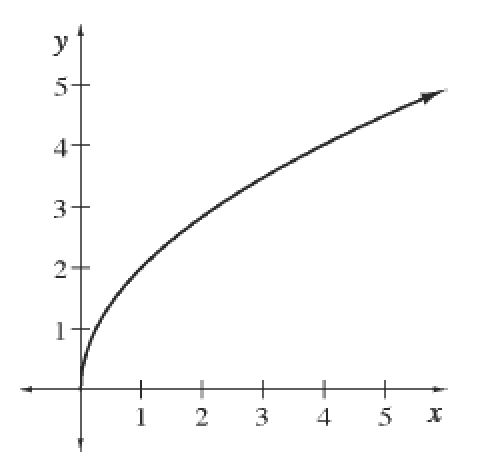### Home > APCALC > Chapter 3 > Lesson 3.3.4 > Problem3-131

3-131.

The graph of $y = f(x)$ is shown at right. Use the graph to list the following values in ascending (increasing) order:

$0, f^\prime(1), f ^\prime(4),\frac { f ( 3 ) - f ( 1 ) } { 3 - 1 }$

$f ^\prime(1)$ means the slope at . Is the slope at $x = 1$ positive, negative or zero? How does this compare with the slope at $x = 4$?

$f ^\prime\left(4\right)$ means the slope at $x = 4$. Is the slope at $x = 4$ positive, negative or zero? How does it compare with the slope at $x = 1$?

$\frac{f(3)-f(1)}{3-1}$

means the average rate of change between $x = 3$ and $x = 1$.

Graphically, this can be seen as the slope of the secant line. Is that slope positive, negative or zero? How does it compare with the slopes at $x = 1$ and $x = 4$, and with the value of $0$?

Looking at the graph from left to right, it is increasing always. The slopes start steep and, as $x \rightarrow \infty$, get smoother.Use the eTool below to help solve the problem.
Click the link at right for the full version of the eTool: Calc 3-131 HW eTool